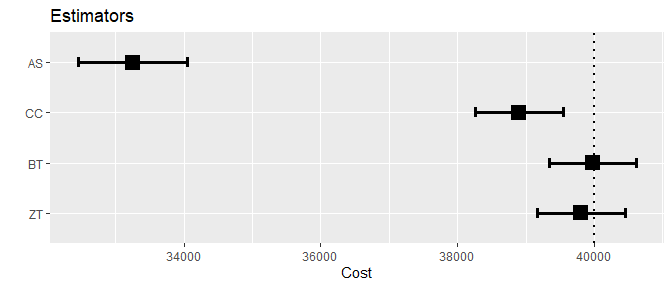# Simulation study: Bias and coverage of the estimators

The ccostr package includes a function to simulate data. We can use it to e.g test the accaracy of the estimates and coverage of confidence intervals. First we load the necessary packages.

library(ccostr)
library(ggplot2)
library(knitr)
library(parallel)
library(msm)

If we use the settings of the simulations described in Lin et al. (1997), it can be shown the true mean cost over 10 years for data simulated with a uniform survival distribution is 40000, while the mean cost for exponentially distributed survival is 35956. Using the simCostData function to simulate data from these distributions, with either light (~25%) or heavy (~40%) censoring, we test the performance of the estimators in the ccostr package.

## Single simulation

For a single simulation with n=1000 individuals with a uniform survival distribution and light censoring we obtain the following results:

set.seed(123)
sim <- simCostData(n = 1000, dist = "unif", censor = "light", cdist = "exp", L = 10)
est <- ccmean(sim$censoredCostHistory) est #> ccostr - Estimates of mean cost with censored data #> #> Observations Individuals FullyObserved Limits TotalTime MaxSurvival #> N 4606 1000 751 9.994045 4100.745 9.994045 #> #> Estimate Variance SE 0.95LCL 0.95UCL #> AS 33248.09 164564.3 405.6653 32452.98 34043.19 #> CC 38901.97 106221.2 325.9160 38263.17 39540.76 #> BT 39979.64 106327.1 326.0784 39340.52 40618.75 #> ZT 39804.74 107538.3 327.9304 39161.99 40447.48 #> #> Mean survival time: 4.94 With SE: 0.1 As seen from both the result tables above and the plot below, estimates are closer to the true value when using the BT and ZT estimators. Both the CC and AS estimators miss the true value, with especially large margins for the AS estimator. plot(est) + geom_hline(yintercept = 40000, linetype = "dotted", size = 1)## Repeated simulations for bias and coverage We now test the bias and coverage of the estimator through more extensive simulations. We perform 1000 simulations with 1000 individuals, for four different scenarios: Uniform and exponential survival functions, with either light or heavy sensoring.  nSim <- 1000 nYears <- 10 indv <- 1000 # increating individuals increases computing time exponential ## true mean for unif is 40000 and exp is 35956 unif_light <- lapply(1:nSim, function(x) simCostData(n = indv, dist = "unif", censor = "light", cdist = "exp", L = nYears)) unif_heavy <- lapply(1:nSim, function(x) simCostData(n = indv, dist = "unif", censor = "heavy", cdist = "exp", L = nYears)) exp_light <- lapply(1:nSim, function(x) simCostData(n = indv, dist = "exp", censor = "light", cdist = "exp", L = nYears)) exp_heavy <- lapply(1:nSim, function(x) simCostData(n = indv, dist = "exp", censor = "heavy", cdist = "exp", L = nYears)) For the calculation of the estimates we use parralization to speed up the process. We make an implicit cluster and use it for computations. Be aware, this might still take quite some time if run on a normal desktop or laptop computer. nCores <- parallel::detectCores() - 1 cl <- makeCluster(nCores) clusterExport(cl = cl, c("unif_light", "unif_heavy", "exp_light", "exp_heavy")) invisible(clusterEvalQ(cl = cl, {library(dplyr) library(ccostr) library(data.table) library(survival)})) est_unif_light <- parLapply(cl, unif_light, function(x) ccmean(x$censoredCostHistory, L = 10))
est_unif_heavy <- parLapply(cl, unif_heavy, function(x) ccmean(x$censoredCostHistory, L = 10)) est_exp_light <- parLapply(cl, exp_light, function(x) ccmean(x$censoredCostHistory, L = 10))
est_exp_heavy  <- parLapply(cl, exp_heavy,  function(x) ccmean(x\$censoredCostHistory, L = 10))
stopCluster(cl)

### Results

The results are pasted together to create a presentable table:

results_unif_light <- do.call(rbind, lapply(est_unif_light, function(x) x[]))
results_unif_heavy <- do.call(rbind, lapply(est_unif_heavy, function(x) x[]))
results_exp_light  <- do.call(rbind, lapply(est_exp_light,  function(x) x[]))
results_exp_heavy  <- do.call(rbind, lapply(est_exp_heavy,  function(x) x[]))
results_true <- data.frame("unif_light" = 40000,
"unif_heavy" = 40000,
"exp_light"  = 35956,
"exp_heavy"  = 35956)
results_bias <- data.frame("unif_light" = (colMeans(results_unif_light)),
"unif_heavy" = (colMeans(results_unif_heavy)),
"exp_light"  = (colMeans(results_exp_light)),
"exp_heavy"  = (colMeans(results_exp_heavy)))
results <- rbind(results_true, results_bias)
row.names(results) <- c("true_mean", colnames(results_unif_light))

results_bias <- cbind(round(results[,c(1,2)] - 40000,2),
round(results[,c(3,4)] - 35956,2))

cov_unif_light <- do.call(rbind, lapply(est_unif_light, function(x) ifelse(x[][5,] >= 40000 & x[][4,] <= 40000, 1, 0)))
cov_unif_heavy <- do.call(rbind, lapply(est_unif_heavy, function(x) ifelse(x[][5,] >= 40000 & x[][4,] <= 40000, 1, 0)))
cov_exp_light  <- do.call(rbind, lapply(est_exp_light,  function(x) ifelse(x[][5,] >= 35956 & x[][4,] <= 35956, 1, 0)))
cov_exp_heavy  <- do.call(rbind, lapply(est_exp_heavy,  function(x) ifelse(x[][5,] >= 35956 & x[][4,] <= 35956, 1, 0)))
results_coverage <- data.frame("unif_light" = (colMeans(cov_unif_light, na.rm = T)),
"unif_heavy" = (colMeans(cov_unif_heavy, na.rm = T)),
"exp_light"  = (colMeans(cov_exp_light,  na.rm = T)),
"exp_heavy"  = (colMeans(cov_exp_heavy,  na.rm = T)))
load(system.file("extdata", "results.Rdata", package = "ccostr"))
kable(results)
unif_light unif_heavy exp_light exp_heavy
true_mean 40000.00 40000.00 35956.00 35956.00
simulation_mean 39998.70 40008.91 35958.00 35967.19
AS 33079.31 29189.39 30929.59 27988.97
CC 38960.44 38183.94 35616.21 35291.55
BT 39993.85 40012.95 35960.69 35964.20
ZT 39981.40 39986.85 36001.38 36038.10

### Bias

Taking the average of all estimates subtracted from the true mean reveals bias estimate. As expected we see a smaller bias when using estimators that take the censoring into account. However, contrary to the results from Zhao and Tian (2001) bias is slightly higher for the ZT estimator than the BT estimator.

kable(results_bias)
unif_light unif_heavy exp_light exp_heavy
true_mean 0.00 0.00 0.00 0.00
simulation_mean -1.30 8.91 2.00 11.19
AS -6920.69 -10810.61 -5026.41 -7967.03
CC -1039.56 -1816.06 -339.79 -664.45
BT -6.15 12.95 4.69 8.20
ZT -18.60 -13.15 45.38 82.10

### Coverage

Similarly, the ratio of times the confidence intervals overlaps the true mean gives a coverage estimate of the estimators. For both the BT and ZT estimators we see coverages close to 95% for all scenariors indicating a good estimation of the 95% confidence intervals.

kable(results_coverage, digits = 3)
unif_light unif_heavy exp_light exp_heavy
AS 0.000 0.000 0.000 0.000
CC 0.128 0.000 0.788 0.491
BT 0.942 0.946 0.938 0.958
ZT 0.947 0.959 0.937 0.955